Question

# Aluminum (Al) has a density (d) of 2.70 g/cm3and crystallizes in a face-centered cubic (fcc) structure....

Aluminum (Al) has a density (d) of 2.70 g/cm3and crystallizes in a face-centered cubic (fcc) structure. What is the unit cell edge length? Select one: a. 2.47 × 10-3pm. b. 40.0 pm. c. 405 pm. d. 321 pm. e. 255 pm.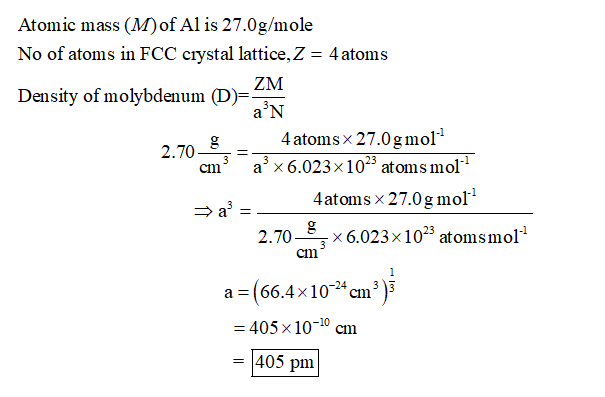#### Earn Coins

Coins can be redeemed for fabulous gifts.

Similar Homework Help Questions
• ### Aluminum crystallizes with a face-centered-cubic unit cell. The radius of an Al atom is 143 pm....

Aluminum crystallizes with a face-centered-cubic unit cell. The radius of an Al atom is 143 pm. Calculate the density of solid crystalline Al in g/cm3.

• ### Metal x crystallizes in a face-centered cubic (close-packed) structure. The edge length of the unit cell...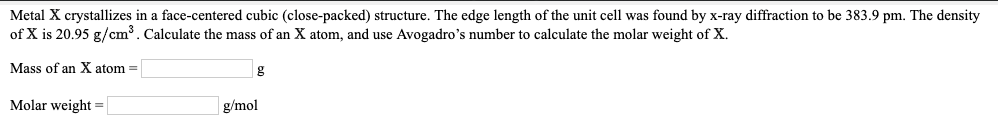Metal x crystallizes in a face-centered cubic (close-packed) structure. The edge length of the unit cell was found by x-ray diffraction to be 383.9 pm. The density of x is 20.95 . Calculate the mass of an x atom, and use Avogadro’s number to calculate the molar weight of Metal X crystallizes in a face-centered cubic (close-packed) structure. The edge length of the unit cell was found by x-ray diffraction to be 383.9 pm. The density of X is 20.95...

• ### Gold crystallizes in a face-centered cubic structure. What is the edge length of the unit cell...

Gold crystallizes in a face-centered cubic structure. What is the edge length of the unit cell if the atomic radius of gold is 144 pm?407 pm204 pm288 pm333 pm

• ### Aluminum (atomic mass 26.98 g/mol) crystallizes in a face-centered cubic unit cell. In addition, aluminum has...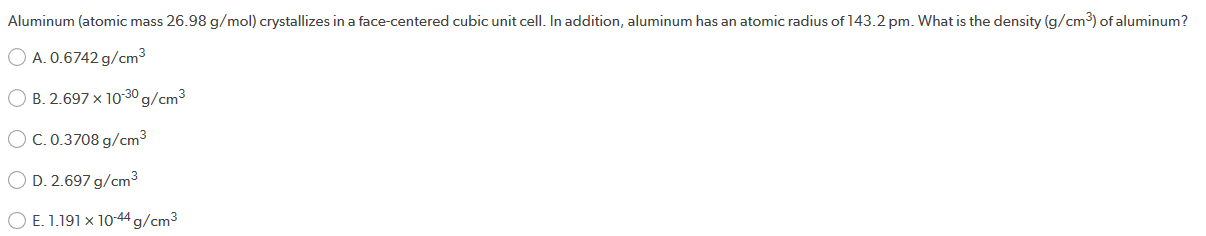Aluminum (atomic mass 26.98 g/mol) crystallizes in a face-centered cubic unit cell. In addition, aluminum has an atomic radius of 143.2 pm. What is the density (g/cm3) of aluminum? O A. 0.6742 g/cm3 B. 2.697 x 10-30 g/cm3 OC.0.3708 g/cm3 OD. 2.697 g/cm3 O E. 1.191 x 10-44 g/cm3

• ### Determine the radius of in Al atom (in pm) if the density of the aluminum is...

Determine the radius of in Al atom (in pm) if the density of the aluminum is 2.71g/cm3. Aluminum crystallizes in a face centered cubic structure with an edge length of 2 square root 2 r

• ### Lead has a radius of 154 pm and crystallizes in a face-centered cubic unit cell. What...

Lead has a radius of 154 pm and crystallizes in a face-centered cubic unit cell. What is the edge length of the unit cell? A. 35 pm B. 1232 pm C. 54 pm D. 436 pm

• ### has a density of 12.41 g/cm and crystallizes with the face-centered cubic unit ly show all...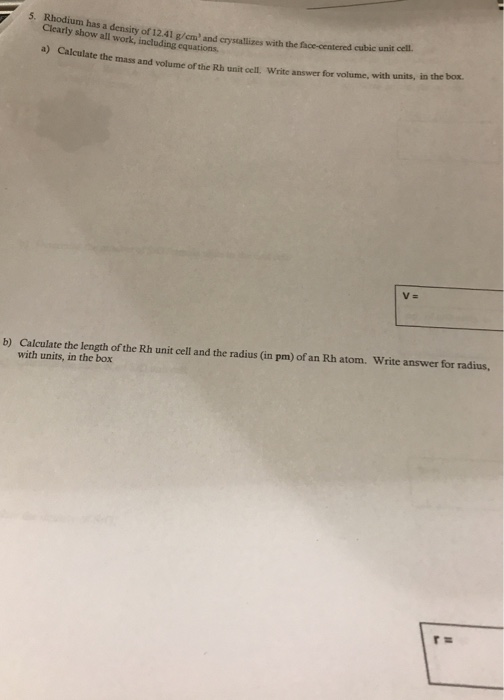has a density of 12.41 g/cm and crystallizes with the face-centered cubic unit ly show all work, including equations mass and volume of the Rb unit cell. Write answer for volume, with units, in the box. V- Calculate the length of the Rh unit cell and the radius (in pm) of an Rh atom. with units, in the box Write answer for radius, b)

• ### Need the answer of #3 and #4 A and B 3. a-Polonium crystallizes in a body-centered...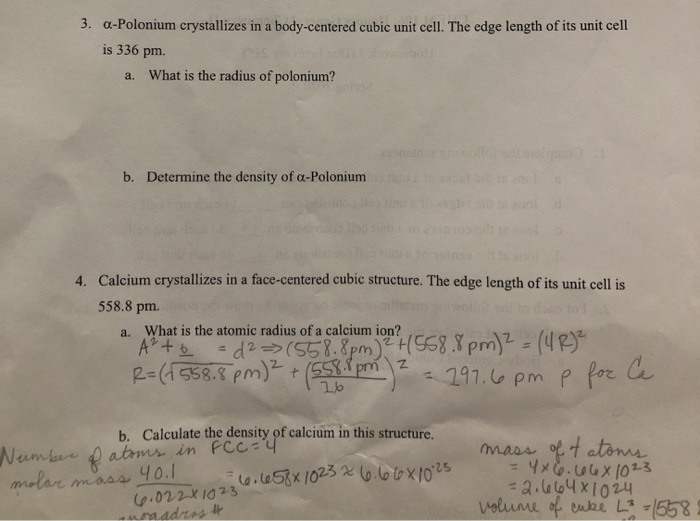Need the answer of #3 and #4 A and B 3. a-Polonium crystallizes in a body-centered cubic unit cell. The edge length of its unit cell is 336 pm. a. What is the radius of polonium? b. Determine the density of a-Polonium da listabaid 4. Calcium crystallizes in a face-centered cubic structure. The edge length of its unit cell is 558.8 pm. a. What is the atomic radius of a calcium ion? AP + 6 - 12 >(558.8pm)2 558.pm)2 =...

• ### gold (Au) crystallizes in a face centered cubic unit cell with an edge length of 407pm....

gold (Au) crystallizes in a face centered cubic unit cell with an edge length of 407pm. calculate the density (g/cm^3)

• ### Aluminum crystallizes in a face-centered cubic lattice. If the atomic radius of the aluminum is 143...

Aluminum crystallizes in a face-centered cubic lattice. If the atomic radius of the aluminum is 143 pm. What is the density of Aluminum? please show steps